Economics Tutors | Micro, Macro & Econometrics Tuitions | EconTutors

# Economics Tutor Berlin – Microeconomics, Macroeconomics, Econometrics

### Work with our Economics tutor in Berlin for all economics and finance coursework.The graph above represents a typical Cobb-Douglas utility function given by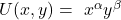. Higher level of utility is presented by a higher utility function such that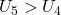, meaning the consumer is better off at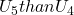.

Recall that total derivative of a function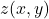is given by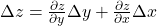#### Axioms of Preferences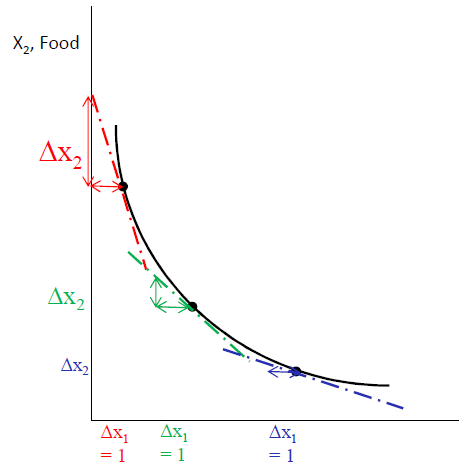Monotonicity (non-satiation)
– We are talking about goods and not bads >> More is better!
– Consider two bundles X and Y. If Y has at least as much of both goods, and more of one, then

(y1,y2)≥(x1,x2)

Convexity
– Averages are better than extremes (or at least not worse)
– An average of two bundles on the same indifference curve will be (at least weakly) preferred

Completeness
– The consumer can always compare/rank bundles.

Continuous
– If X is preferred to Y, and there is a third bundle Z which lies within a small radius of Y, then X will be preferred to Z.

#### Marginal Rate of Substitution

• So the slope of an indifference curve is equal to the ratio of marginal utilities (will become important later).
• If indifference curves are convex, so that the MRS is diminishing:

–We can notice a few things about the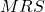function. As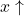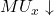, conversely as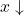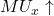. What happens to the MRS in this case? As a consumer moves along the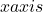(1)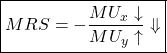–As we get more q1 each additional unit is worth less to us (so U_1 goes down).

–As we get less q2 each additional unit is worth more to us (so U_2 goes up).

Other popular courses:

Get Started

## See the #1 economics mentoring platform in action

Request a demo